# DAV Class 8 Science Chapter 4 Question Answer – Force and Pressure

The DAV Class 8 Science Book Solutions Pdf and DAV Class 8 Science Chapter 4 Question Answer – Force and Pressure are essential study tools for DAV public school students in Class 8.

## DAV Class 8 Science Ch 4 Question Answer – Force and Pressure

### DAV Class 8 Science Ch 4 Solutions – Force and Pressure

Something To Know

A. Fill in the blanks:

Question 1.
For a force to come into play, two concerned bodies must ________ with each other.
interact

Question 2.
To draw water from a well, we have to ________ the rope.
pull

Question 3.
An unbalanced force, acting on a moving object, may change its ________ and/ or its ________.
velocity, direction

Question 4.
In the game of cricket, the hails’ fall when the ball strikes them. This is an example of a/an ________ force.
electrostatic

Question 5.
The force, responsible for raising our body hair, when we try to take off a synthetic (terylene or polyster) shirt in dry weather conditions, is an ________ force.
unbalanced

Question 6.
The atmospheric pressure ________ as we move towards higher altitudes.
decreasesB. State True or False for the following statements:

1. The effect of a force may change by changing the direction of the applied force.
True

2. A force has to be specified both in terms of its magnitude as well as its direction.
True

3. Frictional force is an example of a non-contact force.
False

4. A gas filled balloon moves up. The upward force, acting on it is smaller than the force of gravity, i. e. the downward acting force.
False

5. We can reduce the pressure of a given force by increasing the area over which the force acts.
True

6. Inside a bottle filled with water, pressure is least at the bottom and the greatest at the surface of water.
False

C. Tick the correct option

Question 1.
When two forces, applied on an object, are equal and opposite, then the forces-
(a) May move the object
(b) May stop a moving object
(c) May move the object and also cause a change in its shape.
(d) Do not move the object but may cause a change in its shape.
(d) Do not move the object but may cause a change in its shape.

Question 2.
The following are the names of the well known force
A. Gravitational force
B. Muscular force
C. Magnetic force
D. Frictional force
Out of these, the ‘contact forces’ are the forces labelled as:
(a) A and B
(b) B and C
(c) B and D
(d) C and D
(c) B and D

Question 3.
Rohini is pushing a box towards the west direction with a force of magnitude F.
To help her move the box faster in the same direction, her friend should-
(a) Push the box in the east direction with a force of magnitude F.
(b) Pull the box in the west direction with a force of magnitude F.
(c) Push the box in the west direction with a force of any magnitude.
(d) Push the box in the east direction with a force of any magnitude.
(c) Push the box in the west direction with a force of any magnitude.Question 4.
A container is filled with water as shown in the figure below.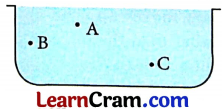Which of the following statements is correct about the pressure of water in the container?
(a) Pressure at A > pressure at B > pressure at C
(b) Pressure at C > pressure at B > pressure at A
(c) Pressure at A = pressure at B = pressure at C
(d) Pressure at A < pressure at B > pressure at C
(b) Pressure at C > pressure at B > pressure at A

Question 5.
An empty metal bottle has a to be placed on a table so that is exerts the maximum pressure on the surface of table?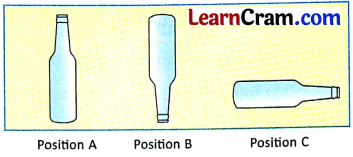For this, the bottle needs to be placed in the position (s) labelled as:
(a) Position A
(b) Position B
(c) Position C
(d) Position A and C
(b) Position B

Question 6.
When we press the bulb of a dropper, with its nozzle kept under water, air in the dropper is seen to escape in the form of bubbles. If we then release the pressure on the bulb, water gets filled in the dropper. The rise of water in the dropper is due to:
(a) Pressure of water in the container
(b) Weight of the bulb
(c) Gravity of the earth
(d) Atmospheric pressure
(d) Atmospheric pressure

Question 7.
The length, breadth and height, of a given rectangular box, are 100 cm, 50 cm and 20 cm respectively. This box is kept on ground in three different ways, one by one. Which of the following statements is correct about the pressure exerted by this box on the ground?
(a) The pressure exerted is maximum when the ‘length’ and ‘height’ sides form the base.
(b) The pressure exerted is maximum when the ‘length’ and ‘breadth’ sides form the base.
(c) The pressure exerted is maximum when the “breadth’ and ‘height’ sides form the base.
(d) The pressure exerted remains the same irrespective of which of its ‘sides’ form the base.
(c) The pressure exerted is maximum when the “breadth’ and ‘height’ sides form the base.

D. Answer the following questions in brief:

Question 1.
In the following situations, identify the agent exerting the force. Also, state the effect of the force in each case.
(a) Squeezing a piece of lemon between the fingers to extract its juice.
Agent of force: fingers, Effect: change in shape

(b) A person diving into a swimming pool.
Agent of force: Person’s muscles, Effect: change in motion

(c) A labourer moving a loaded cart.
Agent of force: Labourer, Effect: change in motion of cart

(d) A car coming to rest once its engine is switched off.
Agent of force: Friction from road, Effect: change in motion

(e) An athlete making a high jump to clear a bar kept at a certain height.
Agent of force: Muscles, Effect: change in motionQuestion 2.
An object experiences a net non-zero force. Is it possible for the object to move with a constant speed along a straight line?
When an object experiences a net non-zero force, it is not possible for the object to move with a constant speed along a straight line.

Question 3.
Distinguish between
(a) Balanced and unbalanced forces.
When net force on an object is zero then it is called balanced force. When the net force on an object is non-zero, it is called unbalanced force.

(b) Contact and non-contact forces.
When a force needs to contact an object to exert its influence it is called contact force. When a force can exert its influence without touching or contacting with the object, it is called non-contact force. Muscular force is an example of contact force. Magnetic force is an example of non-contact force.

Question 4.
When we throw a ball upwards, what happens to its speed while it is going up? Give reason for your answer.
When we throw a ball upwards, its speed gradually decreases. It happens because of force of gravity is working in opposite direction of the motion of the ball.

Question 5.
The figure shows a man with a parachute.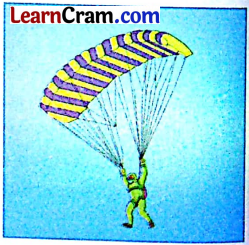(a) Name the force which is responsible for his downward motion. Is it a contact force or a non-contact force?
Force of gravity. It is a non-contact force.

(b) Will the man come down with the same speed without the parachute? Explain.
Without a parachute, the man will come down with faster speed. Air drag works in presence of parachute, and reduces the speed. But negligible air drag works in absence of parachute.

Question 6.
How would the pressure (exerted by a force on an area) change if
(a) The area is doubled keeping the thrust constant?
Pressure will become half.

(b) Thrust is doubled keeping the area constant?
Pressure will become double.

Question 7.
When will a diver ‘experience a greater pressure’; 100 cm below the surface of water or 200 cm below the surface of water? Justify your answer.
Pressure of fluid increases with increase in depth. Hence, the diver will experience a greater pressure at 200 cm below the surface of water.Question 1.
Define force. Is it necessary for the two bodies to be in direct contact for a force to exist between them? Explain with examples.
Ans. A push or a pull is called force. Contact (direct or indirect) between two bodies is necessary for contact force to exist between them. No contact is necessary for non-contact force to act between them. A horse makes a contact with the cart by means of harness; to apply muscular force on the cart. A magnet can pull iron nail even from a distance.

Question 2.
State three effects of a force. Give suitable examples.
Three effects of force are as follows:
Force can change the state of motion: When you kick a football, the football comes into motion.
Force can change the direction of motion: When a batsman hits a ball, direction of motion of the ball changes.
Force can change shape: Dough is changed into chapatti because of force applied by a rolling pin.

Question 3.
Give reasons for the following statements:
(a) ‘Snow shoes’ are more effective than ordinary shoes for walking on snow.
Snow shoes have very wide base and thus a larger area on which force is being applied by the feet. Greater area results in less pressure. Hence, a snow shoe is more effective than ordinary shoes for walking on snow.

(b) Porters place a large round piece of cloth on their heads when they carry heavy loads.
The round piece of cloth helps in increasing the area on which force acts. This helps in reducing pressure. It makes it easier to carry the load on the head.

(c) A sharp knife cuts vegetables and fruits more effectively than a blunt knife.
A sharp knife provides smaller area on which the thrust works. This creates more pressure. Hence, it is easier to cut vegetables and fruits with a sharp knife rather than with a blunt knife.

(d) School bags are provided with wide straps to carry them.
Wide straps provide more area. This helps in reducing pressure on shoulder. It becomes comfortable to carry the school bag. Hence, school bags are provided with wide straps.

(e) It is much easier to burst an inflated balloon with a needle than (directly) with the finger.
The tip of the needle has much smellier area than the tip of a finger. So, the tip of the needle creates more pressure than finger. Hence, it is much easier to burst an inflated balloon with a needle than directly with the finger.

(f) Mountaineers may suffer from nose bleeding at high altitudes.
Air pressure reduces as we go to higher altitudes. At higher altitudes, air pressure is less than blood pressure. Due to this, blood starts oozing out of the capillaries in nose.

(g) Airplanes have pressurized cabins for passengers’ safety while flying high in the atmosphere.
Air pressure reduces as we go to higher altitudes. Low air pressure can be highly uncomfortable for air travellers. Hence, airplanes have pressurized cabins for passengers’ safety while flying high in the atmosphere.

Question 4.
Two rods ‘A’ and ‘B’, of the same weight and equal length have different thicknesses. A boy, while playing with them on a beach, held them vertically on the surface of sand as shown in the figure. Which one of them will sink less? Explain.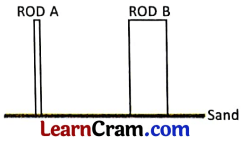We know that pressure varies directly with thrust and inversely with area on which force is applied. For equal amount of force, a smaller area would result in greater pressure. Hence, rod A will sink more while rod B will sink less.

Question 5.
Three holes, A, B and C, are made in an empty can at different levels, one over the other, as shown in the figure. They are (temporarily) closed with an adhesive tape. The can is now filled with water. What will you observe, when the tape (on all of them) is pulled out? Justify your answer.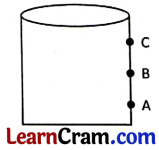Water will start to gush out of three holes. Water from hole A will go to maximum distance because pressure is highest at bottom. Water from hole C will go to the least distance because of the least pressure.

Question 6.
Make two tiny holes of the same size, at equal heights from the bottom in a plastic bottle. Cover them and fill the bottle with water. Let the covers be now removed. State the observations, and the resulting conclusions based on this activity.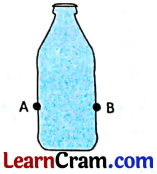Water will start gushing out of two holes. As the holes are at the same height so water will reach same distance. This happens because of same pressure.Question 7.
Discuss in brief the variation in atmospheric pressure with altitude. Do changes in temperature also cause a change in atmospheric pressure?
Atmospheric pressure decreases with increase in altitude. For constant volume, pressure increases with increase in temperature. In other words, at constant volume, pressure is directly proportional to temperature.

Value Based Question

On coming back from school, Anudit asked his mother to explain to him the concepts related to the addition/subtraction of forces acting along the same line. Mother explained to him the reason for adding such forces when they act along the same direction and of subtracting, one from the other, when they act along mutually opposite directions. She went on to say that, in a similar manner, we get an ‘added up and enhanced effect’, when we use our strength, hard work and attention together, for achieving our desired goal.

Question 1.
State the Values’ conveyed by Anudit’s mother.
Combined effect of strength, hard work and focus.

Question 2.
Write down the magnitude and direction of the net force in the following situations:
(a) In tug of war, Team A is applying a force of 100 N along the east direction and Team B is applying a force of 200 N along the west direction.
100 N along west direction

(b) A boy is pushing a box with a force of 100 N towards left by overcoming a force of friction that has a magnitude of 50 N.
50 N towards left

(c) Have a group discussion in which students give examples of ‘combined’ and ‘similarly directed efforts’ of a number of individuals, producing enhanced/better ‘positive results’.
Do it yourself

Something To Do

Question 1.
Design your own barometer to predict weather.
(Use an empty jar, a long necked bottle, coloured water and a marker pen.)
Note the changes in the marked level over a period of a few weeks and ‘predict weather’ on the basis of your observations.
Hint: Higher marked level indicates high air pressure and fine weather, lower marked level indicates low air pressure; this may cause a stormy weather.
Do it yourself

Question 2.
How is pressure related to force and area?
Design an experiment/activity to explain how this inverse relationship, between pressure (due to a given force) and area, is used in daily life.
Do it yourself

Question 3.
Force and pressure are two different concepts though at times we tend to use these two words interchangeably. Collect some pictures, or draw cartoons, to bring out the difference between the two.
Do it yourself### DAV Class 8 Science Chapter 4 Solutions – Force and Pressure Important Questions and Answers

I. Fill in the blanks:

1. A ________ or ________ is called force.
Push, pull

2. The SI unit of force is ________ .
Newton

3. The unit of pressure is ________ .
Pascal

4. Force of friction acts in ________ direction to the direction of motion.
Opposite

5. The earth attracts everything towards itself because of force of ________ .
Gravitation

Question 1.
In which of the following cases, the net of two forces would be non-zero?
(a) When forces are acting in the same direction
(b) When forces are acting in opposite directions
(c) Both ‘a’ and ‘b’
(d) None of these
(c) Both ‘a’ and ‘b’

Question 2.
Which of the following is an example of contact force?
(a) Force applied by a magnet
(b) Force applied by a charged body
(c) Gravitational force
(d) Force applied by a horse on a cart
(d) Force applied by a horse on a cart

Question 3.
Which device is used for measuring atmospheric pressure?
(a) Barometer
(b) Thermometer
(c) Milemeter
(d) Speedometer
(a) BarometerQuestion 4.
In case of which of these, the atmospheric pressure would be very low?
(a) On a hot sunny day
(b) On a cold day
(c) On a stormy day
(d) During night
(c) On a stormy day

Question 5.
In which of the following cases, the direction of motion can change?
(a) When force is being applied in the direction of motion
(b) When force is being applied at an angle to the direction of motion
(c) When force is being applied parallel to the direction of motion
(d) All of these
(b) When force is being applied at an angle to the direction of motion

Question 1.
What are the effects of force on state of motion?
Ans. Force can change the state of motion of an object. If an object is in the state of rest, it can be changed into state of motion by force. If an object is in the state of motion, it can be changed into state of rest by force. Force also changes the direction of motion and rate of motion.

• If a force is applied on a body in the direction of motion, it increases the speed.
• If a force is applied on a body in the opposite direction of motion, it decreases the speed.
• If a force acts at an angle to the direction of motion, it changes the direction of motion.

Question 2.
What do you understand by balanced forces?
When the net of different forces acting on a body is zero they are called balanced forces.

Question 3.
What do you understand by unbalanced forces?
When the net of different forces acting on a body is not zero they are called unbalanced forces.

Question 4.
Explain contact forces and their types.
When a force can be applied only through physical contact with a body it is called contact force. Following are some examples of contact forces:

• Muscular Force: Force applied by the muscles of humans or animals is called muscular force. When a horse is pulling a cart, it is applying muscular force.
• Frictional Force: When two surfaces are in motion in relation to each other, a force acts between them and opposes the motion. This force is called force of friction or frictional force. The direction of force of friction is opposite to the direction of motion.Question 5.
What do you understand by pressure?
When a force is applied perpendicular to a given surface area, this force is called thrust. Thrust acting on a unit area of a surface is called pressure.
Pressure = $$\frac{\text { Thrust }}{\text { Surface area over which it acts }}$$
A bigger surface area means less pressure for the given thrust, compared to a smaller surface area.

IV. Projects:

Question 1.
Make a list of five activities in daily life in which you need to increase pressure for accomplishing a task.